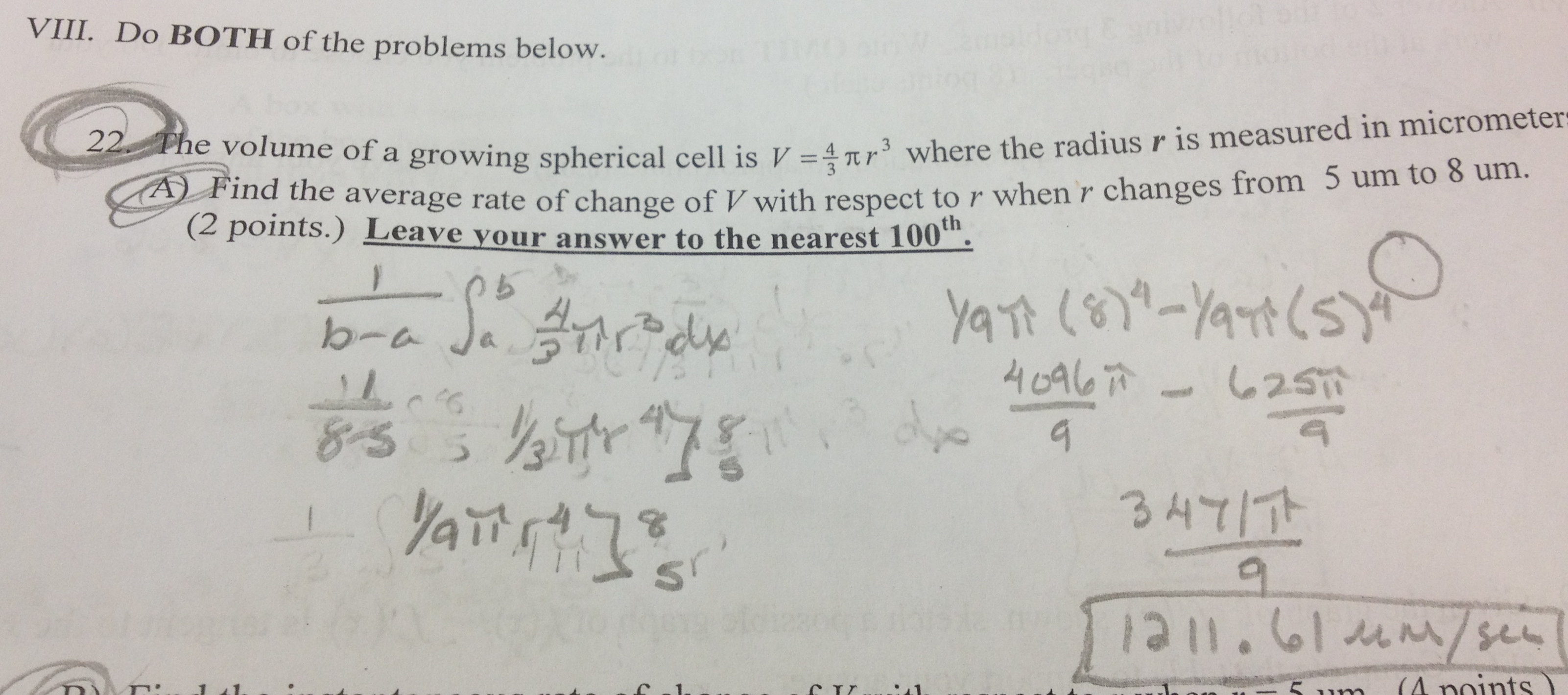Categories

# Rate of change of a volume of a growing sphere

There were two parts to this calculus problem, and both parts are below. Time to sharpen your Calc skills, folks:Calculus pros: what’s going on here? Where did the student go right?  Where did she go wrong?  How would you help?

## 6 replies on “Rate of change of a volume of a growing sphere”louisesays:

Did we have one like this before? I say have the student calculate ending and beginning volumes , then do slope. Then lead from divide by x to divide by$/delta x$
The student knows to do “something” and it’s calculus, so they did integration, presumably because that’s what they did last week. The student does know how to integrate, but unfortunately has no idea what it means in real life.
Maybe it comes from – to accumulate a rate, integrate…? So the student does not understand what accumulate means.louisesays:

I did that wrong, didn’t I?$\delta x$Barrysays:

This is exactly the same problem as http://mathmistakes.org/?p=239, just different work. My answer is still the same. Both students know more than just how to do “something” — they both know the formula for “the average value of a continuous function over a closed interval.”:

http://mathcentral.uregina.ca/QQ/database/QQ.09.00/esther2.html

The average value of V’ over the interval [5,8] is defined to be$\frac{1}{8-5} \int_{5}^8 V' dr$

The Fundamental Theorem of Calculus shows that this is the same thing as the average rate of change of V over the interval [5,8], which is what the problem requested. So there are two methods to do this — use the formula for average rate of change of V over [5,8] or compute instead the average value of V’ over [5,8]

The present student calculated, unfortunately, the average value of V over the interval [5,8], not the average value of V’. And unfortunately for the previous student, he/she did take V’ but did not then compute an antiderivative of V’ while computing the average value of V’ over [5,8]. Even if that student had correctly taken this anti-derivative, I don’t trust with what is written that the student would understand their computation is equivalent to computing the average rate of change of V.

I agree with everything Barry said, and I want to think about how to cure these kinds of mistakes. To me it makes it look like the student has no idea what’s going on, and is just mindlessly executing procedures and using formulas that they’ve memorized.

Maybe I should require my students to draw a graph of what they’re doing, label the axes properly, and mark relevant points, sketch a tangent line if you calculate a derivative, shade an area if you calculate an integral?

I suppose they could still do the same work we see here, but if they’re shading an area under the V vs r graph, at least there’s some hope that they would realize that this doesn’t make any sense. If the question has “rate of change”, there should be a line with a slope being calculated.Barrysays:

I agree that requiring a diagram of some sort can give both teacher and student better knowledge of what the student understands. Here, however, I think it is also imperative in this case that the language confusion be resolved. They see the word “average”, and immediately start in the solution using the first meaning of “average” that enters their heads. It has never occurred to these students that there are two usages, “average rate of change” and “average value of a function” (as well as other usages in probability!), and that anytime they see “average” they must think carefully about which is meant before exploring the problem and its solution.

Whenever I cover average value of a function, I stress this confusion and give some problems assessing their ability to distinguish the different meanings and proceed appropriately. As a result, I see fewer solutions like the one above.

I think your awareness of these common confusions is vital to teaching these topics well. Maybe that will be one of the best results of this blog — more teachers aware of the common difficulties and prepared in their teaching to make students confront and resolve them!